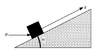# Trunk up an Incline

bartman

## Homework Statement

A trunk of mass m = 1.8 kg is pushed a distance d = 62 cm up an incline with an angle of inclination θ = 24.0° by a constant horizontal force P = 375 N (see figure). The coefficient of kinetic friction between the trunk and the incline is 0.19.
Figure: img144.imageshack.us/img144/8295/prob13ani7.gif

A) Calculate the work done on the trunk by the applied force P.
B) Calculate the work done on the trunk by the frictional force.
C) Calculate the work done on the trunk by the gravitational force.

sin θ = y/h
cos θ = x/h
N*μk=Fk

## The Attempt at a Solution

A)
cos(θ)*F*h
cos(24°)*375N*0.62m = 212J

B)
N*μk=Fk
(sin(24°)*375N + cos(24°)*1.8kg*9.8m/s/s)*.62m*.19 = 19.9J

C)
This is where I am having problems.

#### Attachments

•prob13a.gif
5.1 KB · Views: 821
Last edited: